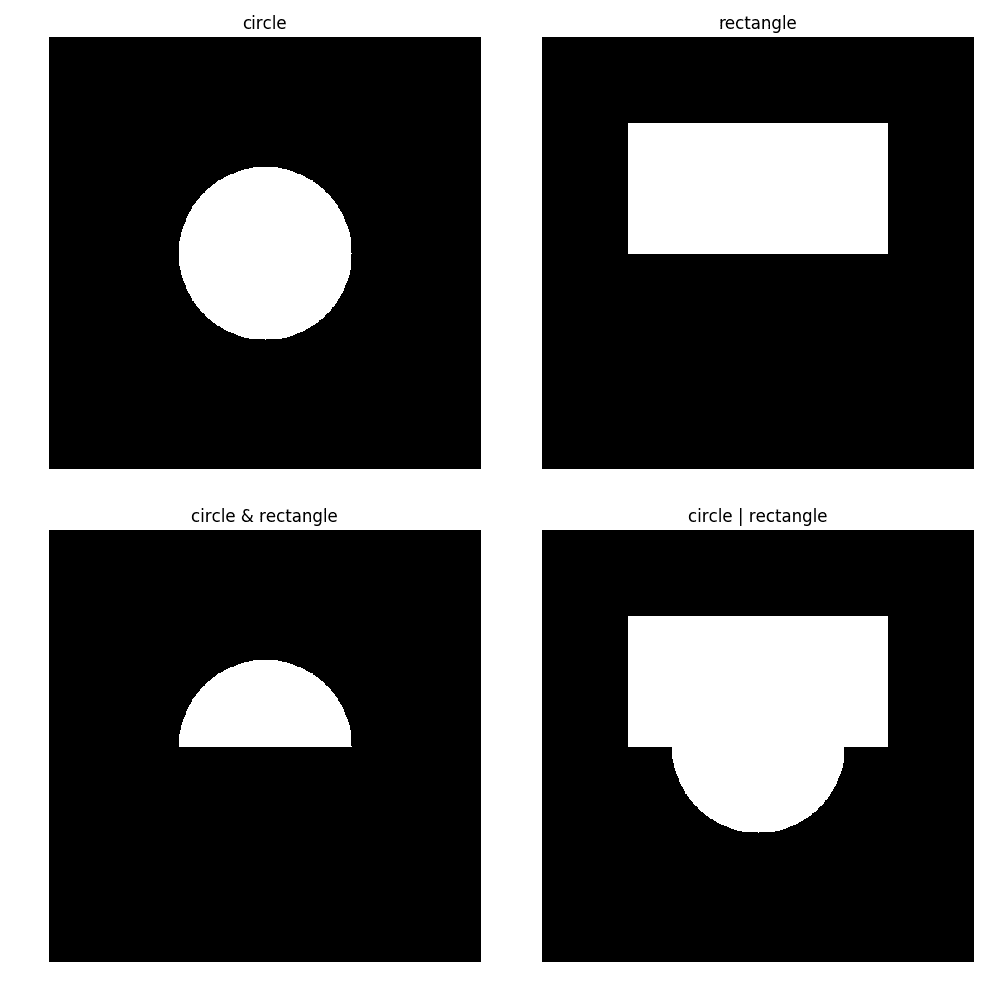# 二、矩阵，颜色和过滤器

• 处理矩阵的创建，填充，访问元素和 ROI
• 在不同的数据类型和缩放值之间转换
• 使用 NumPy 的非图像数据持久化
• 操作图像通道
• 将图像从一种色彩空间转换为另一种色彩空间
• 伽玛校正和逐元素算数
• 均值/方差图像归一化
• 计算图像直方图
• 均衡图像直方图
• 使用高斯，中值和双边过滤器消除噪声
• 使用 Sobel 过滤器计算梯度图像
• 创建和应用自己的过滤器
• 使用实值 Gabor 过滤器处理图像
• 使用离散傅里叶变换从空间域转到频域（并返回）
• 为图像过滤在频域中操作图像
• 使用不同阈值处理图像
• 形态运算符
• 二进制图像 - 图像遮罩和二进制操作

# 操作步骤

1. 导入所有必要的模块：
import cv2, numpy as np

1. 创建一个特定形状的矩阵，并将其填充为255作为值，该矩阵应显示以下内容：
image = np.full((480, 640, 3), 255, np.uint8)
cv2.imshow('white', image)
cv2.waitKey()
cv2.destroyAllWindows()

1. 创建一个矩阵并为每个像素的颜色设置单独的值，以将我们的矩阵变为红色：
image = np.full((480, 640, 3), (0, 0, 255), np.uint8)
cv2.imshow('red', image)
cv2.waitKey()
cv2.destroyAllWindows()

1. 用零填充矩阵以使其为黑色：
image.fill(0)
cv2.imshow('black', image)
cv2.waitKey()
cv2.destroyAllWindows()

1. 接下来，将某些单个像素的值设置为白色：
image[240, 160] = image[240, 320] = image[240, 480] = (255, 255, 255)
cv2.imshow('black with white pixels', image)
cv2.waitKey()
cv2.destroyAllWindows()

1. 现在，让我们将所有像素的第一个通道设置为255，以使黑色像素变为蓝色：
image[:, :, 0] = 255
cv2.imshow('blue with white pixels', image)
cv2.waitKey()
cv2.destroyAllWindows()

1. 现在，将图像中间垂直线上的像素设置为白色：
image[:, 320, :] = 255
cv2.imshow('blue with white line', image)
cv2.waitKey()
cv2.destroyAllWindows()

1. 最后，将特定区域内所有像素的第二个通道设置为255
image[100:600, 100:200, 2] = 255
cv2.imshow('image', image)
cv2.waitKey()
cv2.destroyAllWindows()


# 工作原理

OpenCV 的 Python 界面中的矩阵与 NumPy 数组一起显示。 NumPy 提供了强大而清晰的工具来处理多维矩阵（也称为张量）。 而且，当然，NumPy 支持纯二维矩阵。 这就是为什么我们需要导入其模块。 这就是为什么我们在此秘籍中使用大量np函数的原因。

np.full用于创建矩阵。 它采用以下参数：（高度，宽度，通道）格式的矩阵形状，每个像素（或像素的每个组成部分）的初始值以及像素值的类型。 可以将单个数字作为第二个参数传递-在这种情况下，所有像素值都使用该数字初始化。 同样，我们可以为每个像素元素传递初始编号。

np.fill可帮助您为所有像素分配相同的值-只需传递一个值即可分配为参数。 np.fillnp.full之间的区别在于，第一个不是创建矩阵，而是为现有元素分配值。

:符号还有助于指定矩阵内的某些区域-我们只需要在:之前添加索引，在:之后添加索引（范围的末尾不包含索引）。 例如，image[100:600, 100:200, 2]为我们提供了具有[100, 600]范围内的高度索引，[100, 200]范围内的宽度索引和通道索引2的所有像素。

# 操作步骤

1. 导入所有必需的模块，打开图像，打印其形状和数据类型，然后在屏幕上显示：
import cv2, numpy as np
print('Shape:', image.shape)
print('Data type:', image.dtype)
cv2.imshow('image', image)
cv2.waitKey()
cv2.destroyAllWindows()

1. 使用浮动数据类型元素将我们的图片转换为一张图片：
image = image.astype(np.float32) / 255
print('Shape:', image.shape)
print('Data type:', image.dtype)

1. 通过2缩放图像元素，并裁剪值以将其保持在[0, 1]范围内：
cv2.imshow('image', np.clip(image*2, 0, 1))
cv2.waitKey()
cv2.destroyAllWindows()

1. 将图像的元素缩放回[0, 255]范围，并将元素类型转换为 8 位无符号int
image = (image * 255).astype(np.uint8)
print('Shape:', image.shape)
print('Data type:', image.dtype)

cv2.imshow('image', image)
cv2.waitKey()
cv2.destroyAllWindows()


# 工作原理# 操作步骤

1. 导入所有必要的模块：
import cv2, numpy as np

1. 创建一个具有随机值初始化的矩阵，并打印其属性：
mat = np.random.rand(100, 100).astype(np.float32)
print('Shape:', mat.shape)
print('Data type:', mat.dtype)

1. 使用np.savetxt函数将随机矩阵保存到文件中：
np.savetxt('mat.csv', mat)

1. 现在，从我们刚刚编写的文件中加载它，并打印其形状和类型：
mat = np.loadtxt('mat.csv').astype(np.float32)
print('Shape:', mat.shape)
print('Data type:', mat.dtype)


# 工作原理

NumPy 的savetxtloadtxt函数使您可以存储和加载任何矩阵。 它们使用文本格式，因此您可以在文本编辑器中查看文件的内容。

# 操作步骤

1. 导入所有必需的模块，打开图像，然后输出其形状：
import cv2, numpy as np
print('Shape:', image.shape)

1. 交换红色和蓝色通道并显示结果：
image[:, :, [0, 2]] = image[:, :, [2, 0]]
cv2.imshow('blue_and_red_swapped', image)

cv2.waitKey()
cv2.destroyAllWindows()

1. 向后交换通道，并按不同比例缩放它们以更改图像的色彩：
image[:, :, [0, 2]] = image[:, :, [2, 0]]
image[:, :, 0] = (image[:, :, 0] * 0.9).clip(0, 1)
image[:, :, 1] = (image[:, :, 1] * 1.1).clip(0, 1)
cv2.imshow('image', image)
cv2.waitKey()
cv2.destroyAllWindows()


# 工作原理# 操作步骤

1. 导入所有必要的模块：
import cv2
import numpy as np

1. 加载图像并打印其形状和类型：
image = cv2.imread('../data/Lena.png').astype(np.float32) / 255
print('Shape:', image.shape)
print('Data type:', image.dtype)

1. 将图像转换为灰度：
gray = cv2.cvtColor(image, cv2.COLOR_BGR2GRAY)
print('Converted to grayscale')
print('Shape:', gray.shape)
print('Data type:', gray.dtype)
cv2.imshow('gray', gray)
cv2.waitKey()
cv2.destroyAllWindows()

1. 将图像转换为 HSV 颜色空间：
hsv = cv2.cvtColor(image, cv2.COLOR_BGR2HSV)
print('Converted to HSV')
print('Shape:', hsv.shape)
print('Data type:', hsv.dtype)
cv2.imshow('hsv', hsv)
cv2.waitKey()
cv2.destroyAllWindows()

1. 通过将V通道乘以某个值来增加图像的亮度。 然后将图像转换为 RGB 颜色空间：
hsv[:, :, 2] *= 2
from_hsv = cv2.cvtColor(hsv, cv2.COLOR_HSV2BGR)
print('Converted back to BGR from HSV')
print('Shape:', from_hsv.shape)
print('Data type:', from_hsv.dtype)
cv2.imshow('from_hsv', from_hsv)
cv2.waitKey()
cv2.destroyAllWindows()


# 工作原理# 操作步骤

1. 将图像加载为灰度并将每个像素值转换为[0, 1]范围内的np.float32数据类型：
import cv2
import numpy as np

image = cv2.imread('../data/Lena.png', 0).astype(np.float32) / 255

1. 使用指定的指数值gamma应用每个元素的指数：
gamma = 0.5
corrected_image = np.power(image, gamma)

1. 显示源图像和结果图像：
cv2.imshow('image', image)
cv2.imshow('corrected_image', corrected_image)
cv2.waitKey()
cv2.destroyAllWindows()


# 工作原理# 操作步骤

1. 导入所有必要的模块：
import cv2
import numpy as np

1. 加载图像并将其转换为包含[0,1]范围内的浮点元素的图像：
image = cv2.imread('../data/Lena.png').astype(np.float32) / 255

1. 从每个图像像素中减去平均值以获得零均值矩阵。 然后，将每个像素值除以其标准差即可得到单位方差矩阵：
image -= image.mean()
image /= image.std()


# 操作步骤

1. 导入所有必要的模块：
import cv2
import numpy as np
import matplotlib.pyplot as plt

1. 加载图像并显示它：
grey = cv2.imread('../data/Lena.png', 0)
cv2.imshow('original grey', grey)
cv2.waitKey()
cv2.destroyAllWindows()

1. 计算histogram函数：
hist, bins = np.histogram(grey, 256, [0, 255])

1. 绘制histogram并显示：
plt.fill(hist)
plt.xlabel('pixel value')
plt.show()


# 工作原理

OpenCV 具有其自己的通用函数来计算直方图cv2.calcHist。 但是，在本秘籍中，我们将使用 NumPy，因为在这种特殊情况下，它使代码更简洁。 NumPy 具有特殊函数来计算直方图np.histogram。 例程的参数是输入图像，箱数和箱范围。 它返回一个带有直方图值和 bin 边值的数组。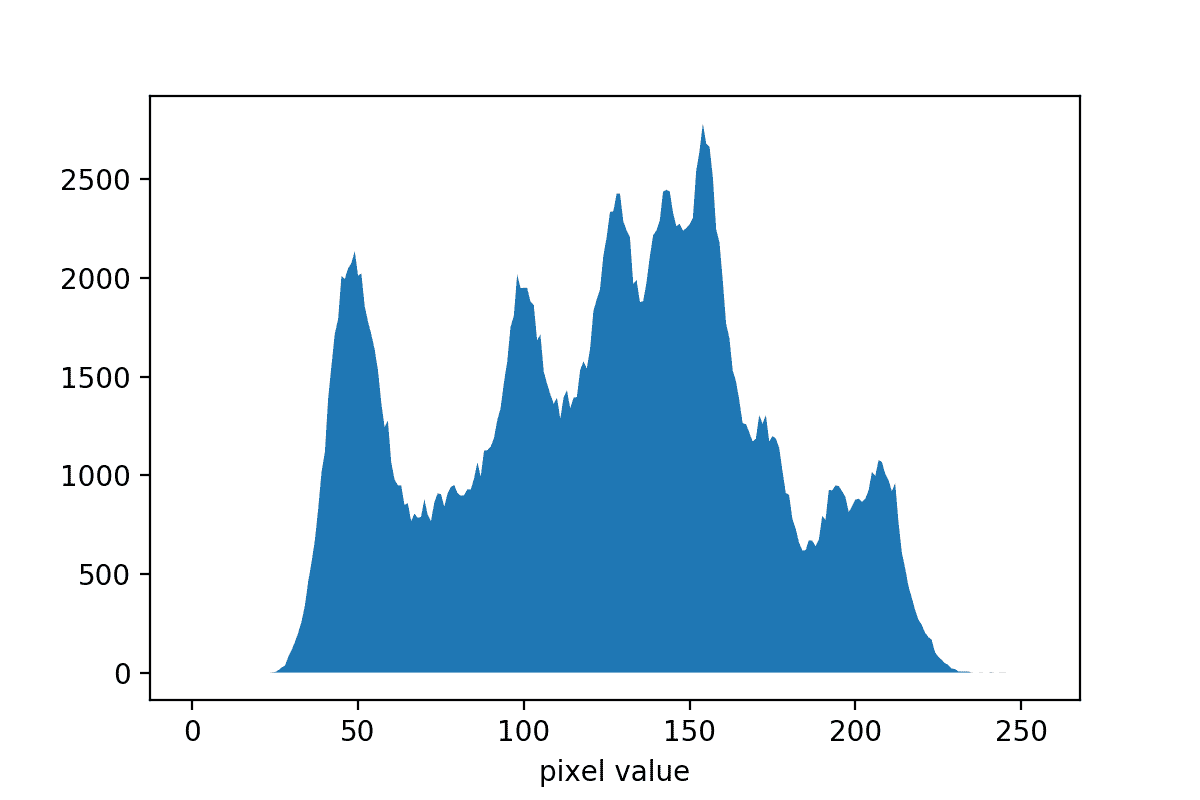# 操作步骤

1. 导入所有必要的模块：
import cv2
import numpy as np
import matplotlib.pyplot as plt

1. 将图像加载为灰度并显示：
grey = cv2.imread('../data/Lena.png', 0)
cv2.imshow('original grey', grey)
cv2.waitKey()
cv2.destroyAllWindows()

1. 均衡灰度图像的直方图：
grey_eq = cv2.equalizeHist(grey)

1. 计算图像的均衡直方图并显示：
hist, bins = np.histogram(grey_eq, 256, [0, 255])
plt.fill_between(range(256), hist, 0)
plt.xlabel('pixel value')
plt.show()

1. 显示均衡的图像：
cv2.imshow('equalized grey', grey_eq)
cv2.waitKey()
cv2.destroyAllWindows()

1. 将图像加载为 BGR 并将其转换为 HSV 颜色空间：
color = cv2.imread('../data/Lena.png')
hsv = cv2.cvtColor(color, cv2.COLOR_BGR2HSV)

1. 均衡 HSV 图像的V通道，并将其转换回 RGB 颜色空间：
hsv[..., 2] = cv2.equalizeHist(hsv[..., 2])
color_eq = cv2.cvtColor(hsv, cv2.COLOR_HSV2BGR)
cv2.imshow('original color', color)

1. 显示均衡的彩色图像：
cv2.imshow('equalized color', color_eq)
cv2.waitKey()
cv2.destroyAllWindows()


# 工作原理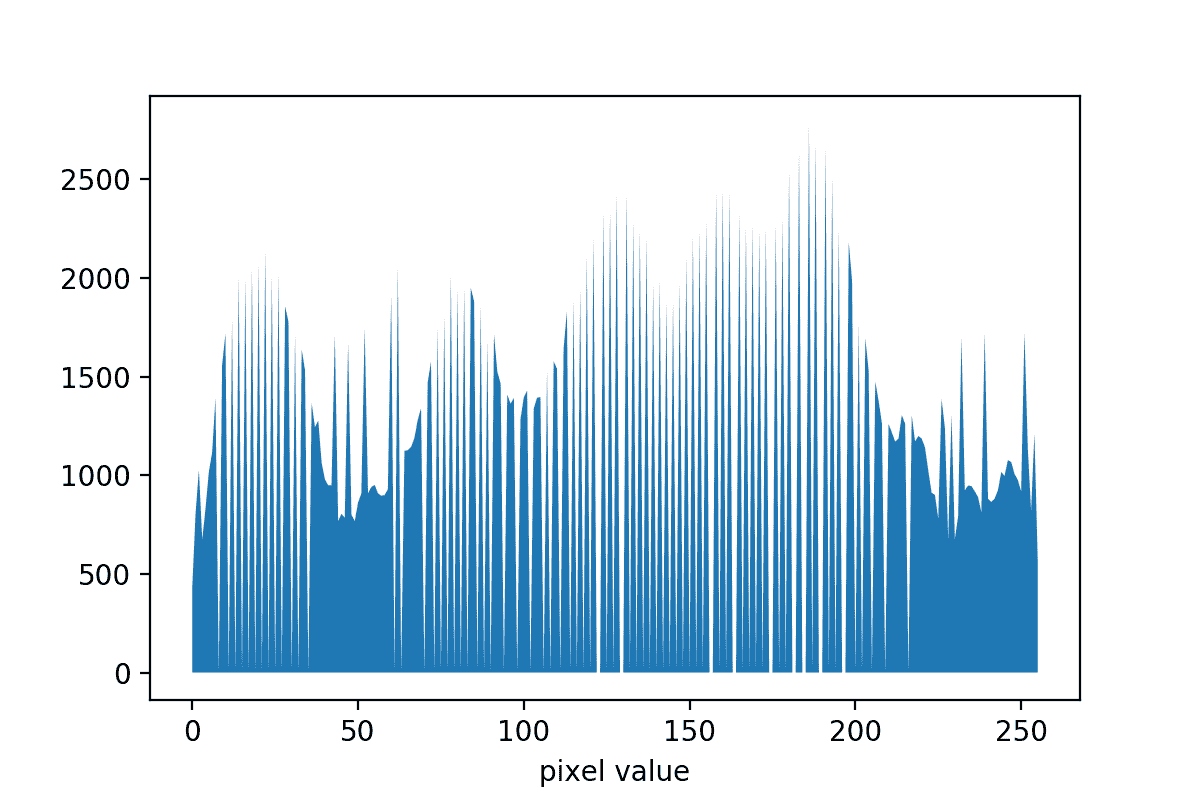# 操作步骤

1. 导入包：
import cv2
import numpy as np
import matplotlib.pyplot as plt

1. 加载图像，将其转换为浮点，然后将其缩小到[0, 1]范围：
image = cv2.imread('../data/Lena.png').astype(np.float32) / 255

1. 通过向每个像素添加随机值来在图像中创建噪点并显示它：
noised = (image + 0.2 * np.random.rand(*image.shape).astype(np.float32))
noised = noised.clip(0, 1)
plt.imshow(noised[:,:,[2,1,0]])
plt.show()

1. GaussianBlur应用于噪点图像并显示结果：
gauss_blur = cv2.GaussianBlur(noised, (7, 7), 0)
plt.imshow(gauss_blur[:, :, [2, 1, 0]])
plt.show()

1. 应用median过滤：
median_blur = cv2.medianBlur((noised * 255).astype(np.uint8), 7)
plt.imshow(median_blur[:, :, [2, 1, 0]])
plt.show()

1. 对我们的图像执行median过滤，并产生噪声：
bilat = cv2.bilateralFilter(noised, -1, 0.3, 10)
plt.imshow(bilat[:, :, [2, 1, 0]])
plt.show()


# 工作原理

cv2.GaussianBlur用于将Gaussian过滤器应用于图像。 此函数获取输入图像，核大小（核宽度，核高度）格式以及沿宽度和高度的标准差。 核大小应为正，奇数。

cv2.bilateralFilter函数提供了双边过滤。 它获取输入图像，窗口大小和颜色以及空间σ值。 如果窗口大小为负，则根据空间σ值计算得出。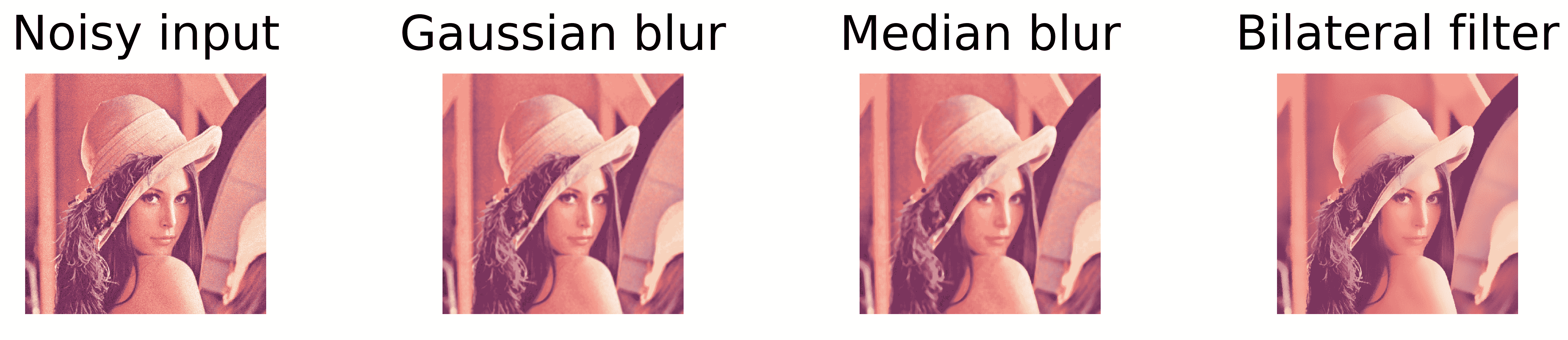# 操作步骤

1. 导入包：
import cv2
import numpy as np
import matplotlib.pyplot as plt

1. 以灰度读取图像：
image = cv2.imread('../data/Lena.png', 0)

1. 使用Sobel运算符计算梯度近似值：
dx = cv2.Sobel(image, cv2.CV_32F, 1, 0)
dy = cv2.Sobel(image, cv2.CV_32F, 0, 1)

1. 可视化结果：
plt.figure(figsize=(8,3))
plt.subplot(131)
plt.axis('off')
plt.title('image')
plt.imshow(image, cmap='gray')
plt.subplot(132)
plt.axis('off')
plt.imshow(dx, cmap='gray')
plt.title(r'$\frac{dI}{dx}$')
plt.subplot(133)
plt.axis('off')
plt.title(r'$\frac{dI}{dy}$')
plt.imshow(dy, cmap='gray')
plt.tight_layout()
plt.show()


# 工作原理

OpenCV 的cv2.Sobel函数使用指定大小的线性过滤器来计算图像梯度近似值。 通过函数参数，您可以确切指定需要计算的导数，应使用的核以及输出图像的数据类型。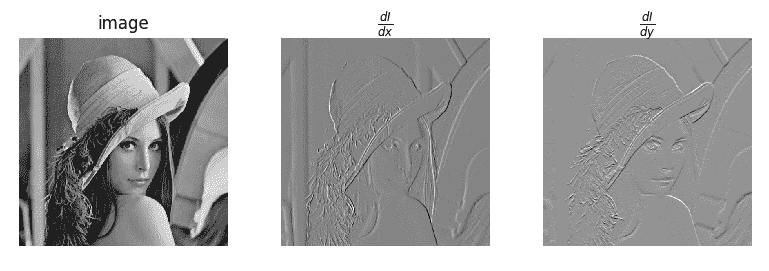# 操作步骤

1. 导入包：
import math
import cv2
import numpy as np
import matplotlib.pyplot as plt

1. 读取测试图像：
image = cv2.imread('../data/Lena.png')

1. 创建一个11x11锐化核：
KSIZE = 11
ALPHA = 2

kernel = cv2.getGaussianKernel(KSIZE, 0)
kernel = -ALPHA * kernel @ kernel.T
kernel[KSIZE//2, KSIZE//2] += 1 + ALPHA

1. 使用我们刚创建的核过滤图像：
filtered = cv2.filter2D(image, -1, kernel)

1. 可视化结果：
plt.figure(figsize=(8,4))
plt.subplot(121)
plt.axis('off')
plt.title('image')
plt.imshow(image[:, :, [2, 1, 0]])
plt.subplot(122)
plt.axis('off')
plt.title('filtered')
plt.imshow(filtered[:, :, [2, 1, 0]])
plt.tight_layout(True)
plt.show()


# 工作原理

OpenCV 的cv2.filter2d函数获取输入图像，输出结果数据类型，OpenCV ID（如果要保留输入图像数据类型，则为 -1）和过滤器核； 然后，对图像进行线性过滤。# 操作步骤

1. 导入包：
import math
import cv2
import numpy as np
import matplotlib.pyplot as plt

1. 读取测试图像为灰度并将其转换为np.float32
image = cv2.imread('../data/Lena.png', 0).astype(np.float32) / 255

1. 构造实值Gabor过滤器核。 规范核，使其具有 L2 单位规范：
kernel = cv2.getGaborKernel((21, 21), 5, 1, 10, 1, 0, cv2.CV_32F)
kernel /= math.sqrt((kernel * kernel).sum())

1. 过滤图像：
filtered = cv2.filter2D(image, -1, kernel)

1. 可视化结果：
plt.figure(figsize=(8,3))
plt.subplot(131)
plt.axis('off')
plt.title('image')
plt.imshow(image, cmap='gray')
plt.subplot(132)
plt.title('kernel')
plt.imshow(kernel, cmap='gray')
plt.subplot(133)
plt.axis('off')
plt.title('filtered')
plt.imshow(filtered, cmap='gray')
plt.tight_layout()
plt.show()


# 工作原理

Gabor过滤器是线性过滤器，其核是用余弦波调制的 2D 高斯调制。 可以使用cv2.getGaborKernel函数获得核，该函数采用诸如核大小，高斯标准差，波方向，波长，空间比和相位之类的参数。 Gabor过滤器有用的领域之一是检测已知方向的边缘。# 操作步骤

1. 导入所需的包：
import cv2
import numpy as np
import matplotlib.pyplot as plt

1. 以灰度读取图像并将其转换为np.float32数据类型：
image = cv2.imread('../data/Lena.png', 0).astype(np.float32) / 255

1. 应用离散傅立叶变换：
fft = cv2.dft(image, flags=cv2.DFT_COMPLEX_OUTPUT)

1. 可视化频谱图：
shifted = np.fft.fftshift(fft, axes=[0, 1])
magnitude = cv2.magnitude(shifted[:, :, 0], shifted[:, :, 1])
magnitude = np.log(magnitude)

plt.axis('off')
plt.imshow(magnitude, cmap='gray')
plt.tight_layout()
plt.show()

1. 将图像从频谱转换回空间表示形式：
restored = cv2.idft(fft, flags=cv2.DFT_SCALE | cv2.DFT_REAL_OUTPUT)


# 工作原理

OpenCV 使用快速傅立叶变换算法（由cv2.dft函数实现）来计算离散傅立叶变换，并将其用于其反向版本（cv2.idft函数）。 这些函数支持可选标志，这些标志指定输出是实数还是复数（分别为标志cv2.DFT_REAL_OUTPUTcv2.DFT_COMPLEX_OUTPUT），以及是否应缩放输出值（使用cv2.DFT_SCALE标志）。 np.fft.fftshift函数以这样的方式移动频谱，即对应于零频率的振幅位于数组的中心，并且更易于解释和进一步使用。# 操作步骤

1. 导入包：
import cv2
import numpy as np
import matplotlib.pyplot as plt

1. 读取图像为灰度并将其转换为np.float32数据类型：
image = cv2.imread('../data/Lena.png', 0).astype(np.float32) / 255

1. 使用离散傅立叶变换将图像从空间域转换为频域：
fft = cv2.dft(image, flags=cv2.DFT_COMPLEX_OUTPUT)

1. 移位 FFT 结果的方式应使低频位于数组的中心：
fft_shift = np.fft.fftshift(fft, axes=[0, 1])

1. 将高频的振幅设置为零，而其他振幅保持不变：
sz = 25

1. 将 DFT 结果移回：
fft = np.fft.ifftshift(fft_shift, axes=[0, 1])

1. 使用逆离散傅里叶逆变换将滤波后的图像从频域转换回空间域：
filtered = cv2.idft(fft, flags=cv2.DFT_SCALE | cv2.DFT_REAL_OUTPUT)

1. 可视化原始图像和过滤后的图像：
plt.figure()
plt.subplot(121)
plt.axis('off')
plt.title('original')
plt.imshow(image, cmap='gray')
plt.subplot(122)
plt.axis('off')
plt.title('no high frequencies')
plt.imshow(filtered, cmap='gray')
plt.tight_layout()
plt.show()


# 工作原理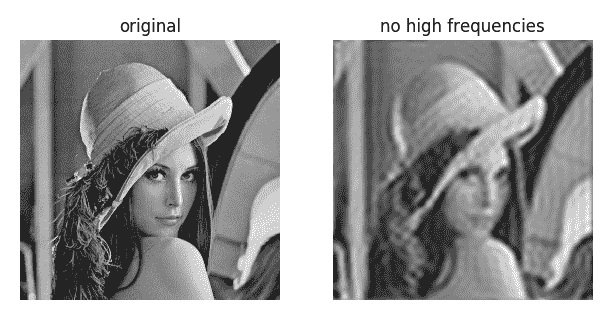# 操作步骤

1. 导入包：
import cv2
import numpy as np
import matplotlib.pyplot as plt

1. 读取测试图像：
image = cv2.imread('../data/Lena.png', 0)

1. 应用一个简单的二进制阈值：
thr, mask = cv2.threshold(image, 200, 1, cv2.THRESH_BINARY)
print('Threshold used:', thr)

1. 应用自适应阈值：
adapt_mask = cv2.adaptiveThreshold(image, 255, cv2.ADAPTIVE_THRESH_MEAN_C,
cv2.THRESH_BINARY_INV, 11, 10)

1. 可视化结果：
plt.figure(figsize=(10,3))
plt.subplot(131)
plt.axis('off')
plt.title('original')
plt.imshow(image, cmap='gray')
plt.subplot(132)
plt.axis('off')
plt.title('binary threshold')
plt.subplot(133)
plt.axis('off')
plt.tight_layout()
plt.show()


# 工作原理

OpenCV 具有许多不同类型的阈值和阈值化方法。 您可以将所有方法分为两类：全局（对所有像素使用相同的阈值）和自适应（对阈值依赖于像素的自适应）。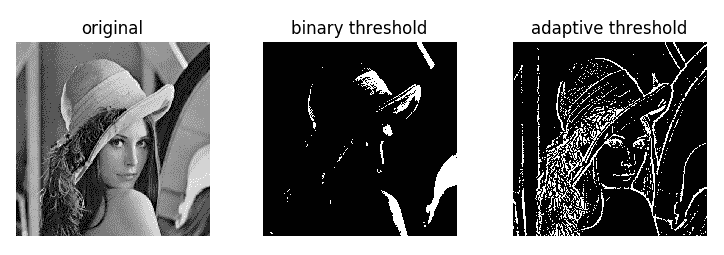# 操作步骤

1. 导入包：
import cv2
import numpy as np
import matplotlib.pyplot as plt

1. 读取测试图像并使用大津的方法构建二进制图像：
image = cv2.imread('../data/Lena.png', 0)
_, binary = cv2.threshold(image, -1, 1, cv2.THRESH_BINARY | cv2.THRESH_OTSU)

1. 使用3x3矩形遮罩施加腐蚀和膨胀 10 次：
eroded = cv2.morphologyEx(binary, cv2.MORPH_ERODE, (3, 3), iterations=10)
dilated = cv2.morphologyEx(binary, cv2.MORPH_DILATE, (3, 3), iterations=10)

1. 使用类似椭圆的5x5结构元素进行 5 次形态学打开和关闭操作：
opened = cv2.morphologyEx(binary, cv2.MORPH_OPEN,
cv2.getStructuringElement(cv2.MORPH_ELLIPSE, (5, 5)),
iterations=5)
closed = cv2.morphologyEx(binary, cv2.MORPH_CLOSE,
cv2.getStructuringElement(cv2.MORPH_ELLIPSE, (5, 5)),
iterations=5)

1. 计算形态梯度：
grad = cv2.morphologyEx(binary, cv2.MORPH_GRADIENT,
cv2.getStructuringElement(cv2.MORPH_ELLIPSE, (5, 5)))

1. 可视化结果：
plt.figure(figsize=(10,10))
plt.subplot(231)
plt.axis('off')
plt.title('binary')
plt.imshow(binary, cmap='gray')
plt.subplot(232)
plt.axis('off')
plt.title('erode 10 times')
plt.imshow(eroded, cmap='gray')
plt.subplot(233)
plt.axis('off')
plt.title('dilate 10 times')
plt.imshow(dilated, cmap='gray')
plt.subplot(234)
plt.axis('off')
plt.title('open 5 times')
plt.imshow(opened, cmap='gray')
plt.subplot(235)
plt.axis('off')
plt.title('close 5 times')
plt.imshow(closed, cmap='gray')
plt.subplot(236)
plt.axis('off')
plt.tight_layout()
plt.show()


# 工作原理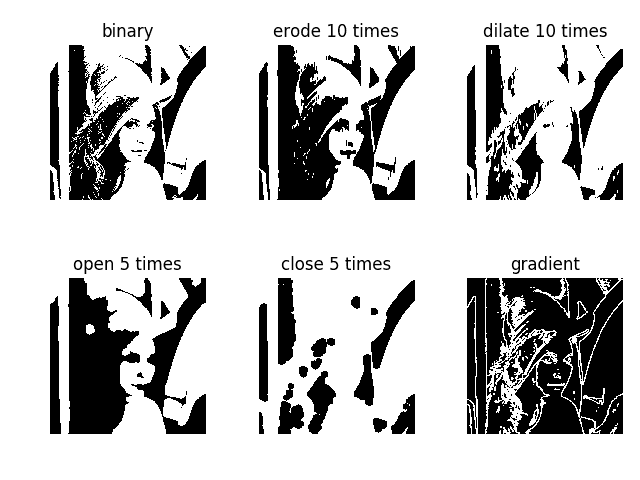# 操作步骤

1. 导入所有包：
import cv2
import numpy as np
import matplotlib.pyplot as plt

1. 创建带有圆形遮罩的二进制图像：
circle_image = np.zeros((500, 500), np.uint8)
cv2.circle(circle_image, (250, 250), 100, 255, -1)

1. 创建一个带有矩形遮罩的二进制图像：
rect_image = np.zeros((500, 500), np.uint8)
cv2.rectangle(rect_image, (100, 100), (400, 250), 255, -1)

1. 使用按位 AND 运算符组合圆形和矩形遮罩：
circle_and_rect_image = circle_image & rect_image

1. 使用按位或运算符组合圆形和矩形遮罩：
circle_or_rect_image = circle_image | rect_image

1. 可视化结果：
plt.figure(figsize=(10,10))
plt.subplot(221)
plt.axis('off')
plt.title('circle')
plt.imshow(circle_image, cmap='gray')
plt.subplot(222)
plt.axis('off')
plt.title('rectangle')
plt.imshow(rect_image, cmap='gray')
plt.subplot(223)
plt.axis('off')
plt.title('circle & rectangle')
plt.imshow(circle_and_rect_image, cmap='gray')
plt.subplot(224)
plt.axis('off')
plt.title('circle | rectangle')
plt.imshow(circle_or_rect_image, cmap='gray')
plt.tight_layout()
plt.show()


# 工作原理